##### Common Core Math For Parents For Dummies with Videos Online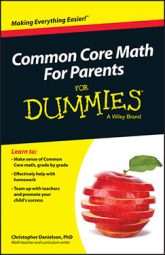In eighth grade Common Core math, students studied similar figures. In high school, they use the properties of similarity to study new things, in particular, trigonometry.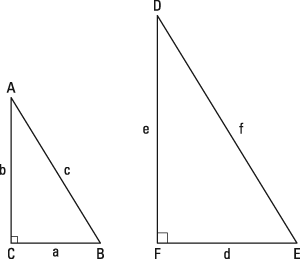Two similar right triangles.

The two triangles in the figure are similar, which means that the measures of corresponding angles in the two triangles are the same, and that their side lengths are proportional. Proportional can refer to one of two relationships in this situation:

• The ratios of corresponding sides (like sides a and d) between the two triangles are all equivalent, so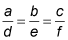This common ratio is sometimes referred to as a scale factor.

• Each ratio of two sides within a triangle is equivalent to the ratio of the corresponding sides in the other triangle, so, for example,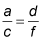These relationships hold for similar triangles in general (and for similar polygons of all kinds, actually). The triangles in the figure have an additional property: They're right triangles. In this case, the ratios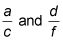are fixed, and their value depends on the measure of the angle at A (and at D, which is the same measure because the triangles are similar). This ratio is sin (A), which you read as sine of A.

Because the ratios of sides of right triangles were figured out long ago and because these ratios are extremely useful for measuring things, the relationships between angle measures and the ratios of sides of the corresponding right triangles have been given names. Sine, cosine, and tangent are the main ratios, but secant, cosecant, and cotangent are similarly defined.

Trigonometry is the study of these functions. The Common Core State Standards don't have a separate set of trigonometry standards. Instead, students study the trigonometry functions as part of their work in geometry — whether in a dedicated geometry course or in an integrated math program across several grades. Either way, a typical precalculus course extends the study of these ratios to angle measure values that don't make sense in right triangles, such as negative numbers and numbers greater than 90 degrees. The Common Core State Standards don't include precalculus standards, so they contain only the right triangle trigonometry that is described here.